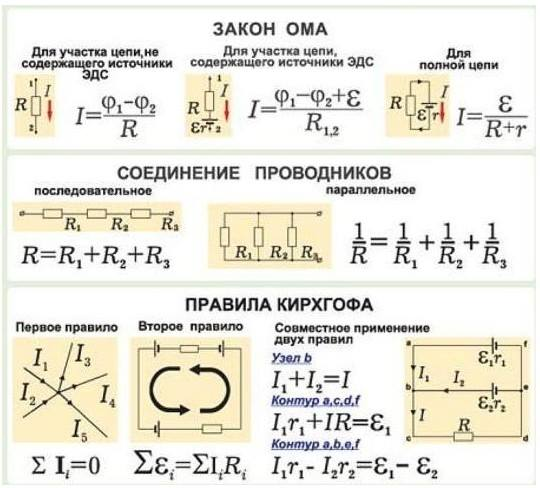This page has been robot translated, sorry for typos if any. Original content here.

# The basics of electrical engineering - the simplest electrical calculationsElectrical engineering - a field of technology related to the receipt, distribution, conversion and use of electrical energy. And also - with the development, operation and optimization of electronic components, electronic circuits and devices, equipment and technical systems.

Under the electrical engineering also understand the technical science, which studies the use of electrical and magnetic phenomena for practical use. Electrical engineering stood out as an independent science from physics at the end of the 19th century.

Currently, electrical engineering as a science includes the following scientific specialties: electrical engineering, TOE, lighting engineering, power electronics. In addition, energy is often referred to the branches of electrical engineering, although a legitimate classification considers energy as a separate technical science. The main difference between electrical engineering and low-current electronics is that electrical engineering studies the problems associated with large-sized power electronic components: power lines, electric drives, while in electronics the main components are computers and other devices based on integrated circuits, as well as integrated circuits themselves schemes. In another sense, in electrical engineering, the main task is the transfer of electrical energy, and in low-current electronics - information.

Ohm's Law is an empirical physical law that determines the relationship of the electromotive force of a source (or electrical voltage) with the strength of the current flowing in a conductor and the resistance of the conductor. Established by Georg Om in 1826 and named in his honor.

Connection of conductors - Serial and parallel connections in electrical engineering are two main ways of connecting elements of an electric circuit. When connected in series, all elements are connected to each other so that the part of the circuit that includes them does not have a single node. In a parallel connection, all elements included in the circuit are connected by two nodes and have no connections with other nodes, if this does not contradict the condition. With a series connection of conductors, the current strength in all conductors is the same. In this case, the total voltage in the circuit is equal to the sum of the voltages at the ends of each of the conductors. In a parallel connection, the voltage drop between the two nodes combining the circuit elements is the same for all elements. In this case, the reciprocal of the total resistance of the circuit is equal to the sum of the quantities inverse to the resistances of the parallel conductors.

The Kirchhoff Rule - (often mistakenly referred to in the literature as the Kirchhoff Laws) - the relationships that apply between currents and voltages in areas of any electrical circuit. Kirchhoff's rules allow you to calculate any electrical circuit of direct, alternating and quasi-stationary current. They are of particular importance in electrical engineering because of their versatility, as they are suitable for solving many problems in the theory of electrical circuits and practical calculations of complex electrical circuits. Applying the Kirchhoff rules to a linear electric circuit allows us to obtain a system of linear equations for currents or voltages, and accordingly, to find the values ​​of currents on all branches of the circuit and all inter-node voltages. Formulated by Gustav Kirchhoff in 1845. The name “Rules” is more correct because these rules are not fundamental laws of nature, but follow from the fundamental laws of conservation of charge and vortex-free electrostatic field (the third Maxwell equation with a constant magnetic field). These rules should not be confused with the two other laws of Kirchhoff in chemistry and physics.

Via FB & wiki